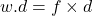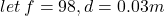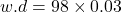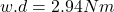## Calculate the workdone by a bricklayer who lifted cement block of mass 10kg from the floor to the height of 3cm g=9.8m​

Question

Calculate the workdone by a bricklayer who lifted cement block of mass 10kg from the floor to the height of 3cm g=9.8m​

in progress 0
7 months 2021-07-29T23:48:52+00:00 1 Answers 11 views 0

The work done is 2.94 Nm.

Explanation:

First, you have to find the force of a brick layer using the formula, Force = mass×gravity :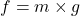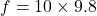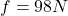Given that the force of a brick layer is 98N. Next, you have to apply work done formula, Work done = force×distance :